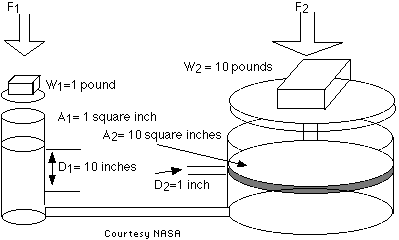QUESTION #526

# How does a hydraulic jack work?

Asked by: Gaurav Kumar

### Answer

Hydraulic jacks and many other technological advancements such as automobile brakes and dental chairs work on the basis of Pascal's Principle, named for Blaise Pascal, who lived in the seventeenth century. Basically, the principle states that the pressure in a closed container is the same at all points. Pressure is described mathematically by a Force divided by Area. Therefore if you have two cylinders connected together, a small one and a large one, and apply a small Force to the small cylinder, this would result in a given pressure. By Pascal's Principle, this pressure would be the same in the larger cylinder, but since the larger cylinder has more area, the force emitted by the second cylinder would be greater. This is represented by rearranging the pressure formula P = F/A, to F = PA. The pressure stayed the same in the second cylinder, but Area was increased, resulting in a larger Force. The greater the differences in the areas of the cylinders, the greater the potential force output of the big cylinder. A hydraulic jack is simply two cylinders connected as described above.
Answered by: R C Rosignol, B.S., Physics Teacher, Pace High School, Brownsville, TX

An enclosed fluid under pressure exerts that pressure throughout its volume and against any surface containing it. That's called 'Pascal's Principle', and allows a hydraulic lift to generate large amounts of FORCE from the application of a small FORCE.

Assume a small piston (one square inch area) applies a weight of 1 lbs. to a confined hydraulic fluid. That provides a pressure of 1 lbs. per square inch throughout the fluid. If another larger piston with an area of 10 square inches is in contact with the fluid, that piston will feel a force of 1 lbs/square inch x 10 square inches = 10 lbs.So we can apply 1 lbs. to the small piston and get 10 lbs. of force to lift a heavy object with the large piston. Is this 'getting something for nothing'? Unfortunately, no. Just as a lever provides more force near the fulcrum in exchange for more distance further away, the hydraulic lift merely converts work (force x distance) at the smaller piston for the SAME work at the larger one. In the example, when the smaller piston moves a distance of 10 inches it displaces 10 cubic inch of fluid. That 10 cubic inch displaced at the 10 square inch piston moves it only 1 inch, so a small force and larger distance has been exchanged for a large force through a smaller distance.
Answered by: Paul Walorski, B.A., Part-time Physics Instructor